# Completing the square - example 2

Complete the square 2x^2-6x+3=0

NOTE:

The coefficient (or number) in front of the x^2  must be a one.

So divide both sides by 2.

(2x^2)/2-(6x)/2+3/2=0/2

x^2-3x+1\1/2=0

Remember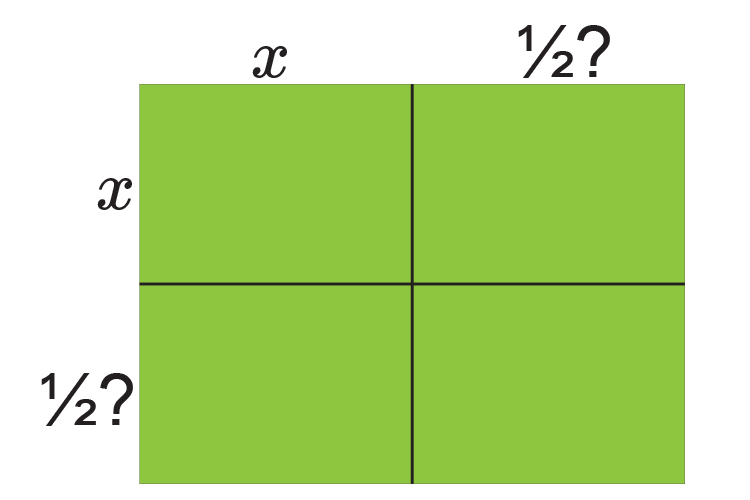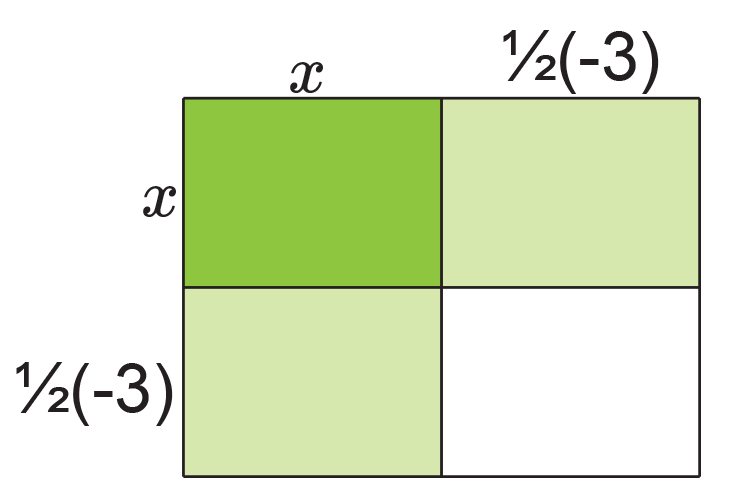Is the same as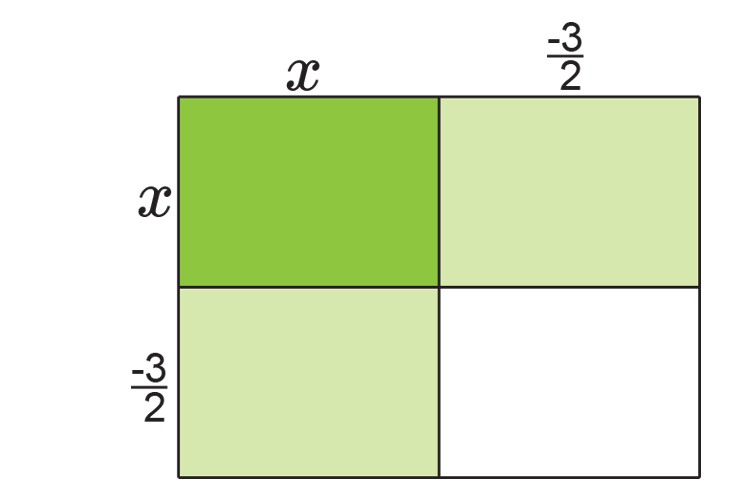Fill in the table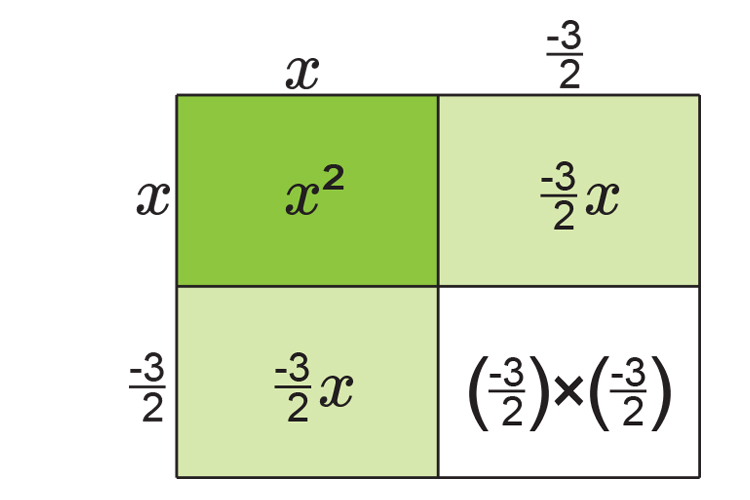Is the same as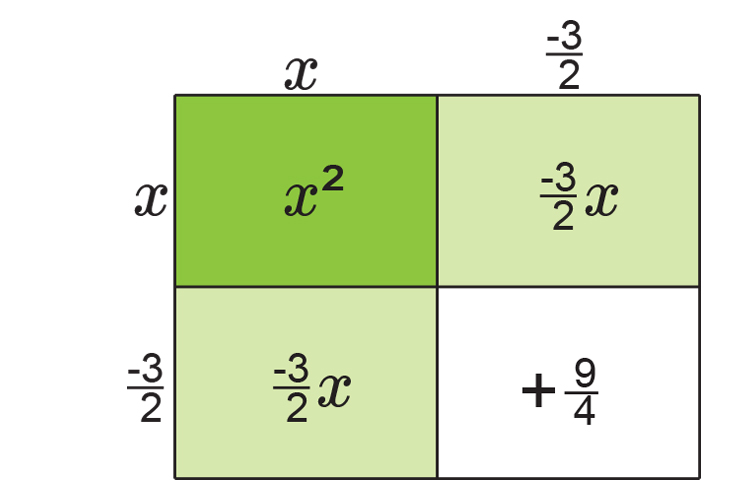NOTE:

This is the same as (x-3/2)^2

If you add up each area you get:

x^2-3/2x-3/2x+9/4

x^2-6/2x+9/4

x^2-3x+9/4

x^2-3x+2\1/4

Originally we had   x^2-3x+1\1/2=0

Now we have          x^2-3x+2\1/4=0

Always plot this on a number line.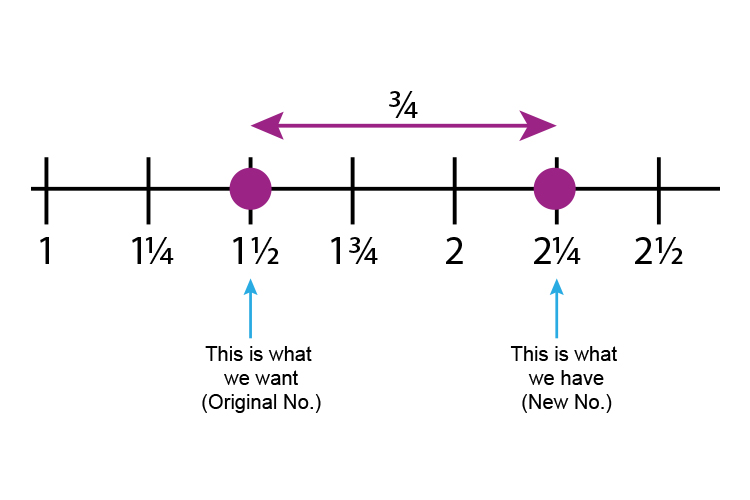Original number -  New number

1\1/2-2\1/4=-3/4   (We need to -3/4 )

So                          x^2-3x+1\1/2=0

Is the same as (x-3/2)^2-3/4=0

Which can now be solved

(x-3/2)^2-3/4=0

(x-3/2)^2=3/4

(x-3/2)=+-sqrt(3/4)

(Don't forget the root of anything can be + or - )

x-3/2=+-sqrt(3/4)

x=3/2+-sqrt(3/4)

x=3/2+sqrt(3/4)   or   x=3/2-sqrt(3/4)

Using calculator

x=1.5+0.866   or   1.5-0.866

x=2.366   or   0.634

### Now check

2x^2-6x+3=0

If x=2.366      2times2.366^2-6times2.366+3=0

11.19-14.19+3=0   Which is correct

If x=0.634      2times0.634^2-6times0.634+3=0

0.804-3.804+3=0   Which is correct

The roots of 2x^2-6x+3=0   are  x=2.366   or   0.634